## Figures index

#### Ehab H. Abdelhay, Fayez W. Zaki, Sherif S. Kishk, Hossam S. Moustafa

International Transaction of Electrical and Computer Engineers System. 2015, 3(1), 19-29 doi:10.12691/iteces-3-1-3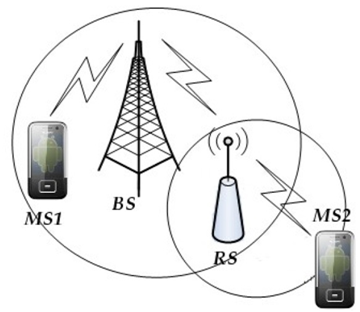• Figure 1. Multi-hop Relay Network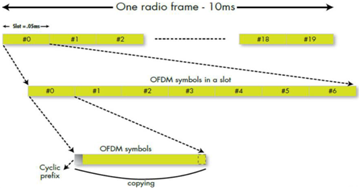• Figure 3. FDD-LTE Frame Structure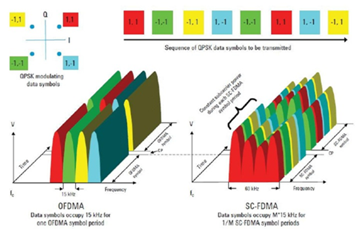• Figure 4. OFDMA and SC-FDMA in LTE• Figure 5. Uplink resource block• Figure 6. RB Scheduling schemes for uplink LTE-A• Figure 7. RS Applications• Figure 8. Non-transparent Mode RS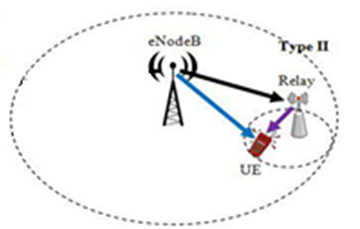• Figure 9. Transparent Mode RS• Figure 10. Amplify and Forward RS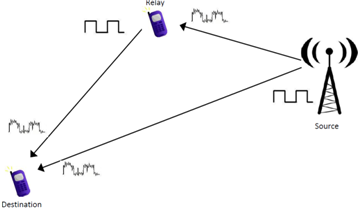• Figure 11. Decode and Forward RS• Figure 12. Relay Station scenarios• Figure 13. RS Link Types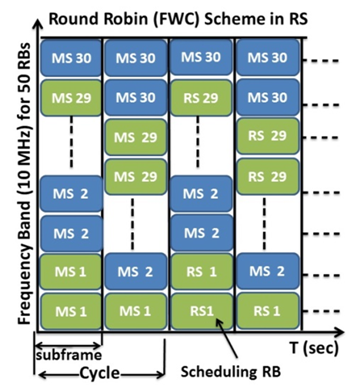• Figure 14. FWC Scheduling with RS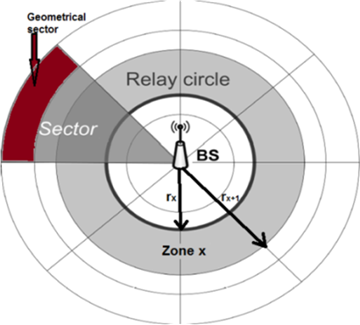• Figure 15. Cell with N-zones and K-sectors• Figure 16. Estimating distance to relay• Figure 17. LTE-A UL FWC using RS• Figure 18. OTFWC cycles using RS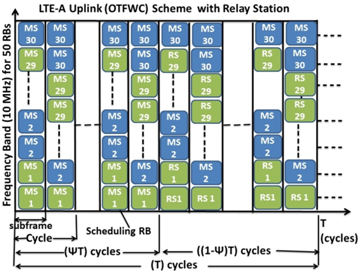• Figure 19. OTFWC transmission using RS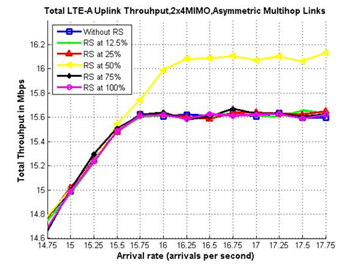• Figure 20. UL throughput, 2X4MIMO, FWC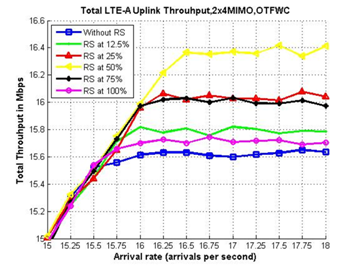• Figure 21. UL Throughput, 2X4MIMO, OTFWC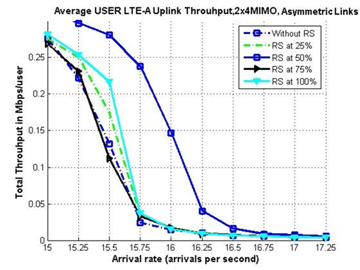• Figure 22. Average User Throughput, 2X4MIMO, FWC• Figure 23. Average User Throughput, 2X4MIMO, OTFWC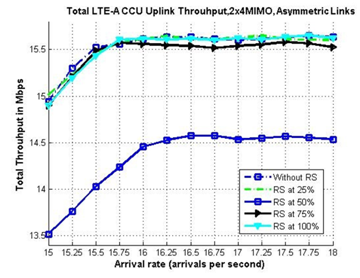• Figure 24. Uplink CCU Throughput, 2x4MIMO, FWC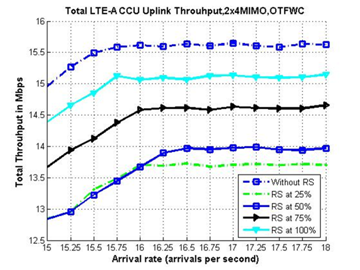• Figure 25. Uplink CCU Throughput, 2x4MIMO, OTFWC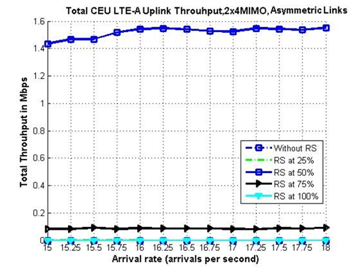• Figure 26. Uplink CEU Throughput, 2x4MIMO, FWC• Figure 27. Uplink CEU Throughput, 2x4MIMO, OTFWC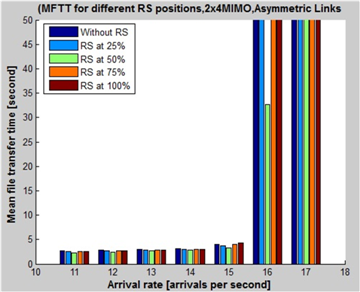• Figure 28. MFTT per cell, 2X4MIMO, FWC• Figure 29. MFTT, 2X4MIMO, OTFWC